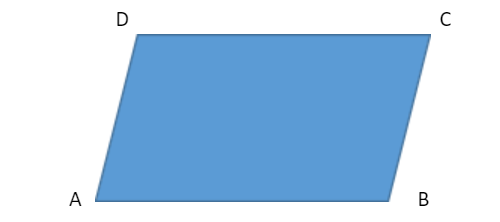Courses
Courses for Kids
Free study material
Free LIVE classes
More# If the given points (1,2), (4,y), (x,6) and (3,5) are the vertices of a parallelogram taken in order. Find x and y.Verified
324.3k+ views
Hint: First name the coordinates of all the vertices of the parallelogram. Find the coordinates of mid-points of the diagonal using the coordinates of the vertices. Since it’s a parallelogram, coordinates bisect each other, hence, equate both coordinates of midpoint obtained from two diagonals and then proceed.Let A(1,2), B(4,y), C(x,6) and D(3,5) are the vertices of a parallelogram ABCD
AC and BD are the diagonals.
O is the mid-point of AC and BD
The coordinates of mid-point are given by $\left[ {\dfrac{{{x_1} + {x_2}}}{2},\dfrac{{{y_1} + {y_2}}}{2}} \right]$
If O is the mid - point of AC, then the coordinates of O are =$\left( {\dfrac{{1 + x}}{2},\dfrac{{2 + 6}}{2}} \right) = \left( {\dfrac{{x + 1}}{2},4} \right)$
If O is the mid-point of BD then coordinates of O are=$\left( {\dfrac{{4 + 3}}{2},\dfrac{{5 + y}}{2}} \right) = \left( {\dfrac{7}{2},\dfrac{{5 + y}}{2}} \right)$
Since both coordinates are of the same point O
∴ $\dfrac{{1 + x}}{2} = \dfrac{7}{2}$
$\Rightarrow 1 + x = 7$
$x = 7 - 1 = 6$
∴$\dfrac{{5 + y}}{2} = 4$
$\Rightarrow 5 + y = 8$
$\Rightarrow y = 8 - 5 = 3$
Hence, x = 6 and y = 3.

Note: Following are the properties of Parallelogram are:
Opposite sides are parallel.
Opposite sides are congruent.
Opposite angles are congruent.
Consecutive angles are supplementary.
Diagonals intersect each other.
Last updated date: 25th May 2023
Total views: 324.3k
Views today: 7.82k